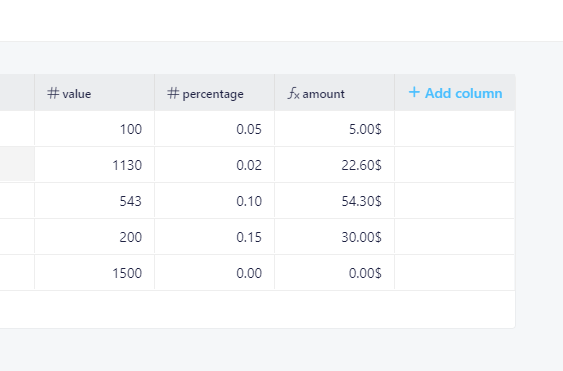# Formula with Zero value returns #Div/0!

#1

I am creating a basic formula to work out the \$ value of a percentage of a invoice
The percentage is usually 10%, 5%, 2% or 0%.

The excel formula would normally be Value * % = % Value for example \$1000 * 10% = \$100

but in Infinity that formula doesn’t work BUT value (\$) / Retention (%) = Retention Value does work.

But when I use Zero as the % value I get #Div/0! as the answer

What am I doing wrong ?

Thanks

0 Likes

#2

Hi @Chris6, first of all - welcome to our community forum, we are happy to see you hereAnd now, regarding the formula, I have actually tested this, I also used “multiply” formula, however - the desired result wasn’t there, same goes with what you have said, if you use dividing option, especially with 0% - it shows - #div/0! as the answer - which is problematic. I’ll put this all in one ticket and report it to our team as a small but frustrating bug.

Workaround I can provide you with is this, instead of using % as the format of the “number” attribute, I would suggest using “decimal” format and writing percentages like this:

1. 5% = 0.05
2. 10% = 0.10
3. 2% = 0.02
4. 0% = 0.00

Now, you can use the basic multiply formula and get the desired number, for example:
100\$ x 0.05 = 5\$Let me know if that helps0 Likes

#3

Hi Marko

Yes came to same conclusion and did the same as you suggest. The divide option only worked on 10% not sure why…